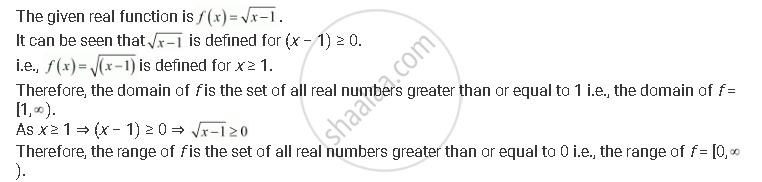CBSE (Arts) Class 11CBSE
Share

# Find the Domain and the Range of the Real Function F Defined by F(X)=Sqrt((X-1)) - CBSE (Arts) Class 11 - Mathematics

ConceptSome Functions and Their Graphs

#### Question

Find the domain and the range of the real function f defined by f(x)=sqrt((x-1))

#### SolutionIs there an error in this question or solution?

#### APPEARS IN

NCERT Solution for Mathematics Textbook for Class 11 (2018 to Current)
Chapter 2: Relations and Functions
Ex. 2.40 | Q: 4 | Page no. 46

#### Video TutorialsVIEW ALL 

Solution Find the Domain and the Range of the Real Function F Defined by F(X)=Sqrt((X-1)) Concept: Some Functions and Their Graphs.
S